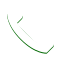## Lesson Five Evaluation

This is your final evaluation. You will need a pass mark of 80% in order to complete this lesson.

## Lesson Three Evaluation

Once again you will need a pass mark of 80% in order to complete this lesson and move on to the lesson titled ‘Additional Lead Generators’.

## Lesson Two Evaluation

In this second evaluation, you will also need a pass mark of 80% in order to complete this lesson and move on to the next lesson which is Appointment Making.

## Lesson Four Evaluation

This is your final evaluation. You will need a pass mark of 80% in order to complete this lesson.

## Lesson One Evaluation

This is your first evaluation. You will need a pass mark of 80% in order to complete this lesson and move to the next.Call Me NOW